# SAS Statistical Business Analysis Using SAS 9: Regression and Modeling v1.0

Page:    1 / 7
Exam contains 103 questions

An analyst fits a logistic regression model to predict whether or not a client will default on a loan. One of the predictors in the model is agent, and each agent serves 15-20 clients each. The model fails to converge. The analyst prints the summarized data, showing the number of defaulted loans per agent. See the partial output below: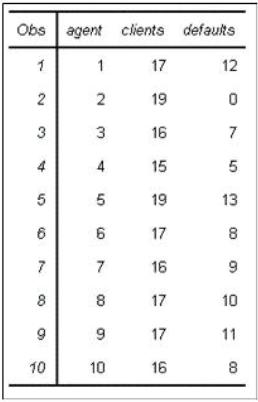What is the most likely reason that the model fails to converge?

• A. There is quasi-complete separation in the data.
• B. There is collinearity among the predictors.
• C. There are missing values in the data.
• D. There are too many observations in the data.

An analyst knows that the categorical predictor, storeId, is an important predictor of the target.
However, store_Id has too many levels to be a feasible predictor in the model. The analyst wants to combine stores and treat them as members of the same class level.
What are the two most effective ways to address the problem? (Choose two.)

• A. Eliminate store_id as a predictor in the model because it has too many levels to be feasible.
• B. Cluster by using Greenacre's method to combine stores that are similar.
• C. Use subject matter expertise to combine stores that are similar.
• D. Randomly combine the stores into five groups to keep the stochastic variation among the observations intact.

Including redundant input variables in a regression model can:

• A. Stabilize parameter estimates and increase the risk of overfitting.
• B. Destabilize parameter estimates and increase the risk of overfitting.
• C. Stabilize parameter estimates and decrease the risk of overfitting.
• D. Destabilize parameter estimates and decrease the risk of overfitting.

An analyst investigates Region (A, B, or C) as an input variable in a logistic regression model.
The analyst discovers that the probability of purchasing a certain item when Region = A is 1.
What problem does this illustrate?

• A. Collinearity
• B. Influential observations
• C. Quasi-complete separation
• D. Problems that arise due to missing values

Refer to the following exhibit: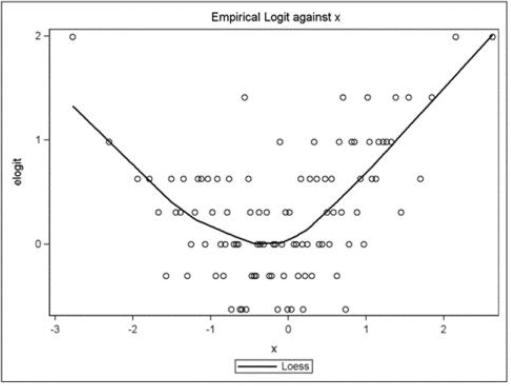What is a correct interpretation of this graph?

• A. The association between the continuous predictor and the binary response is quadratic.
• B. The association between the continuous predictor and the log-odds is quadratic.
• C. The association between the continuous predictor and the continuous response is quadratic.
• D. The association between the binary predictor and the log-odds is quadratic.

This question will ask you to provide a missing option. Given the following SAS program: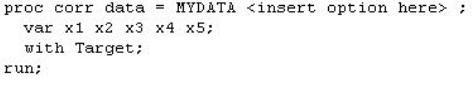What option must be added to the program to obtain a data set containing Pearson statistics?

• A. OUTPUT=estimates
• B. OUTP=estimates
• C. OUTSTAT=estimates
• D. OUTCORR=estimates

A predictive model uses a data set that has several variables with missing values.
What two problems can arise with this model? (Choose two.)

• A. The model will likely be overfit.
• B. There will be a high rate of collinearity among input variables.
• C. Complete case analysis means that fewer observations will be used in the model building process.
• D. New cases with missing values on input variables cannot be scored without extra data processing.

Spearman statistics in the CORR procedure are useful for screening for irrelevant variables by investigating the association between which function of the input variables?

• A. Concordant and discordant pairs of ranked observations
• B. Logit link (log (p/1-p))
• C. Rank-ordered values of the variables
• D. Weighted sum of chi-square statistics for 2x2 tables

A non-contributing predictor variable (Pr > |t| =0.658) is added to an existing multiple linear regression model.
What will be the result?

• A. An increase in R-Square
• B. A decrease in R-Square
• C. A decrease in Mean Square Error
• D. No change in R-Square

The standard form of a linear regression model is: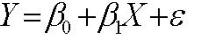Which statement best summarizes the assumptions placed on the errors?

• A. The errors are correlated, normally distributed with constant mean and zero variance.
• B. The errors are correlated, normally distributed with zero mean and constant variance.
• C. The errors are independent, normally distributed with constant mean and zero variance.
• D. The errors are independent, normally distributed with zero mean and constant variance.

Refer to the REG procedure output: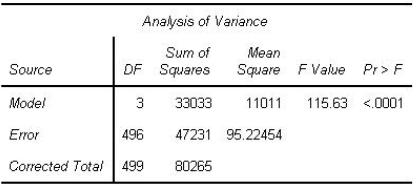Click on the calculator button to display a calculator if needed.

• A. 0.4115
• B. 0.6994
• C. 0.5884
• D. 0.1372

Identify the correct SAS program for fitting a multiple linear regression model with dependent variable (y) and four predictor variables (x1-x4).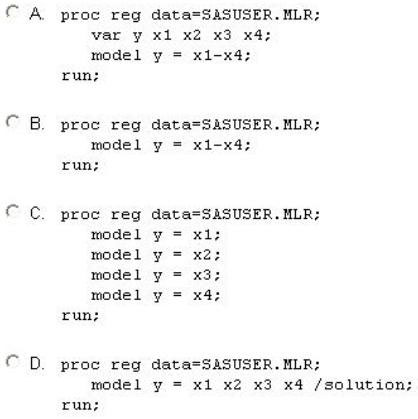• A. Option A
• B. Option B
• C. Option C
• D. Option D

Refer to the REG procedure output: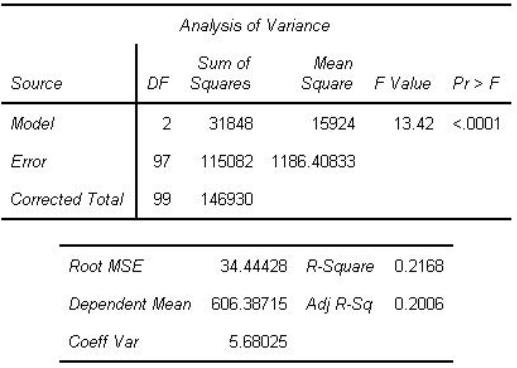An analyst has selected this model as a champion because it shows better model fit than a competing model with more predictors.
Which statistic justifies this rationale?

• A. R-Square
• B. Coeff Var
• D. Error DF

The selection criterion used in the forward selection method in the REG procedure is:

• B. SLE
• C. Mallows' Cp
• D. AIC

Which SAS program will correctly use backward elimination selection criterion within the REG procedure?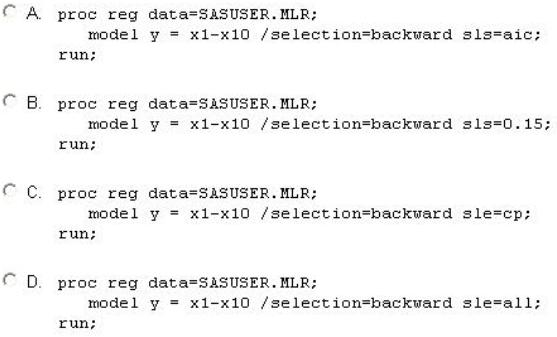• A. Option A
• B. Option B
• C. Option C
• D. Option D